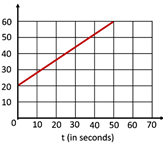science
Easy

Question

# The displacement of the body whose v-t graph is given below is ____ m.Hint:

## The correct answer is: 2000

### Given that,Initial velocity, u = 20 m/sFinal velocity, v = 60 m/sTime taken, t = 50 sThe acceleration was found to be 4/5 m/s2From the second equation of motion, we haves = ut + ½at2Or, s = 20 x 50 + ½ (4/5) (50)2Or, s = 1000 + (2/5) (2500)Or, s = 1000 + 1000Or, s = 2000 mTherefore, the maximum distance covered by the ball is 2000 m.#### With Turito Foundation.#### Get an Expert Advice From Turito.